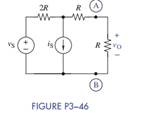Chat Now

# (a) Use the superposition principle to find v O in terms of v S , i S , and R in Figure P3−46....

### (a) Use the superposition principle to find v O in terms of v S , i S , and R in Figure P3−46....

(a) Use the superposition principle to find vO in terms of vS, iS, and R in Figure P3−46.Get solution